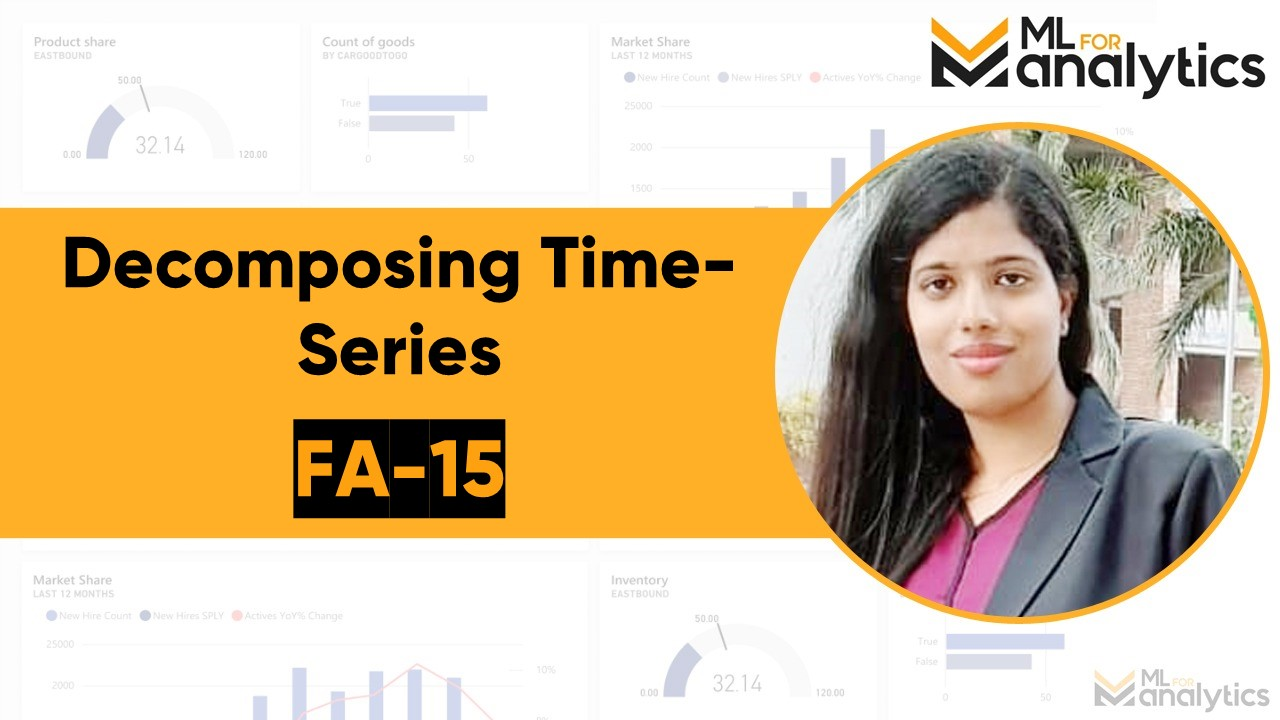# Decomposing time-series: FA15# Decomposing Time-Series

Hi All,

In this tutorial, we will be discussing how to decompose stocks time-series into different components in order to have a good idea about the complexities of the time-series models and how to accurately capture them and account for them in our model.

## Time-series components

Time-series components are of two types:

• Systematic components
• Non-systematic components

### Systematic components

Systematic components can be modeled and described. They are always in tune.

Systematic components are broken down into 3 sub-components:

• Level: It is equal to the mean value of the series.
• Trend: It is defined as change in the successive values of the points of the time-series. This is generally characterized by a slope. If the change in the successive values is positive and the slope is positive, we get increasing trend, else we get decreasing trend.
• Seasonality: It is the deviation from the mean value that happen periodically in less than an year, quarter, month or week.

### Non-systematic components

Non-systematic components cannot be modeled. They constitute of random values and hence, are consist of only noise.

Non-systematic components are broken down into one sub-component:

• Noise: It consist of random variation in data.

## Models for Time-series decomposition

There are two types of models we employ to decompose time-series:

• Multiplicative model

The additive model constitute of the following features:

• The additive model equation is y(t) = level + trend + seasonality + noise
• Linear model: Changes over time are consistent and grow or reduce linearly.
• Seasonality is linear and the frequency and amplitude of the series stay the same over time.

### Multiplicative model

The multiplicative model constitute of the following features:

• The multiplicative model equation is y(t) = level trend seasonality * noise
• Non-linear model: Changes over time are non-consistent and grow or redice non-linearly.
• Seasonality is non-linear and the frequency and amplitude of the series keep on decreasing/increasing over time.

# Decomposing time-series: Code

Let’s start by importing and loading the libraries. We will be importing 4 libraries:

• Pandas for data frame manipulation
• statsmodels.tsa.seasonal for obtaining seasonal_decompose function for decomposing time-series and plotting the time-series decomposition charts. seasonal is the model under tsa (time-series analysis) module of statsmodels package.
In :
```import pandas as pd
import yfinance as yf
from statsmodels.tsa.seasonal import seasonal_decompose
import matplotlib.pyplot as plt
%matplotlib inline
```

• The date range we are selecting varies from 1st Jan 2020 till today.
• For that, let’s fetch today’s date using pandas first and store it in a variable.
• Then we will pass this to the end parameter of download function.
In :
```today = pd.Timestamp('today')
today
```
Out:
`Timestamp('2020-05-29 21:39:05.518364')`
In :
```StockData = yf.download( 'MSFT',
start = '2020-01-01',
end = today,
progress = False)
```

Let’s now see what’s there in the data-set using head function.

In :
```StockData.head()
```
Out:
Open High Low Close Adj Close Volume
Date
2019-12-31 156.770004 157.770004 156.449997 157.699997 156.833633 18369400
2020-01-02 158.779999 160.729996 158.330002 160.619995 159.737595 22622100
2020-01-03 158.320007 159.949997 158.059998 158.619995 157.748581 21116200
2020-01-06 157.080002 159.100006 156.509995 159.029999 158.156342 20813700
2020-01-07 159.320007 159.669998 157.320007 157.580002 156.714310 21634100

Let’s now check the variable statistics using the describe function.

In :
```StockData.describe()
```
Out:
Open High Low Close Adj Close Volume
count 104.000000 104.000000 104.000000 104.000000 104.000000 1.040000e+02
mean 168.610769 171.342308 165.965288 168.736923 168.155711 4.539775e+07
std 13.357310 12.083234 13.805356 13.124158 13.103736 2.082928e+07
min 137.009995 140.570007 132.520004 135.419998 135.043884 1.298303e+07
25% 159.379997 162.887501 157.857498 160.844997 160.286480 3.011120e+07
50% 169.294998 173.089996 165.900002 168.940002 168.449013 3.802435e+07
75% 180.649998 183.597500 177.062500 180.587498 180.085949 5.653928e+07
max 190.649994 190.699997 186.470001 188.699997 187.663330 9.707360e+07

Let’s also see if the data contains any missing values (which is not there, but still checking!).

In :
```StockData.isnull().any()
```
Out:
```Open         False
High         False
Low          False
Close        False
Volume       False
dtype: bool```

No missing values (as expected)! Let’s now start plotting the time-series decomposition chart. We are only interested in Adj Close, so we will obtain a time-series for the same. We will set freq parameter to b or to business days as trading happens on business days only. We will then use the forward fill method to fill N/As.

In :
```data = StockData['Adj Close']
data = data.asfreq('b')
data = data.fillna(method='ffill')
```
In :
```data.head()
```
Out:
```Date
2019-12-31    156.833633
2020-01-01    156.833633
2020-01-02    159.737595
2020-01-03    157.748581
2020-01-06    158.156342
Freq: B, Name: Adj Close, dtype: float64```

Let’s now use data to plot time-series decomposition chart. We will use multiplicative model as in stock trading, nothing is linear, everything is non-linear.

In :
```res = seasonal_decompose(data, model='multiplicative')
fig, ax = plt.subplots(4, 1, figsize=(15,8), sharex=True)
res.observed.plot(ax = ax)

res.trend.plot(ax = ax)

res.seasonal.plot(ax = ax)

res.resid.plot(ax = ax)
```
Out:
`[Text(0, 0.5, 'Residuals'), Text(0.5, 1.0, 'MSFT Adj Close Residuals')]`From above plot, we can see all the four components – Observed (Level), trend, seasonality and residuals (noise) for the Adj Close price.

• We can notice the trend to first increase, reach the top-most point then decrease, reach at the lowest level and then it started increasing again.
• We can also see that the seasonality pattern gets repeated after every week.
• There was very high level of noise in March (second week majorly).
• The noise component was stable during the starting of month of January and end of month of May.

So guys, with this I conclude this tutorial. In the next tutorial, we will be diving deeper into time-series analytics and will apply it on stock data and understand its application in the field of financial analytics. Please don’t forget to subscribe to our channel, ML For Analytics, where we are teaching all this in our videos. Stay tuned!

## One thought on “Decomposing time-series: FA15”

1.Aparna says:

How can we identify that , the
Seasonality is getting repeated weekly ?